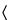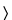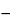846SPECIFIC SURFACE AREA

INTRODUCTION
The specific surface area of a powder is determined by physical adsorption of a gas on the surface of the solid and by calculating the amount of adsorbate gas corresponding to a monomolecular layer on the surface. Physical adsorption results from relatively weak forces (van der Waals forces) between the adsorbate gas molecules and the adsorbent surface of the test powder. The determination is usually carried out at the temperature of liquid nitrogen. The amount of gas adsorbed can be measured by a volumetric or continuous flow procedure.

BRUNAUER, EMMETT AND TELLER (BET) THEORY AND SPECIFIC SURFACE AREA DETERMINATION
Multipoint Measurement
The data are treated according to the Brunauer, Emmett and Teller (BET) adsorption isotherm equation:
1/[Va((PO/P)1)] = [(C1)/(VmC)] × (P/PO) + (1/VmC)(1)
 P = partial vapor pressure of adsorbate gas in equilibrium with the surface at 77.4 K (b.p. of liquid nitrogen), in Pa, Po = saturated pressure of adsorbate gas, in Pa, Va = volume of gas adsorbed at standard temperature and pressure (STP) [273.15 K and atmospheric pressure (1.013 × 105 Pa)], in mL, Vm= volume of gas adsorbed at STP to produce an apparent monolayer on the sample surface, in mL, C = dimensionless constant that is related to the enthalpy of adsorption of the adsorbate gas on the powder sample.
A value of Va is measured at each of not less than three values of P/Po.
Then the BET value
1/[Va((Po/P)1)]
is plotted against P/Po, according to equation (1). This plot should yield a straight line usually in the approximate relative pressure range 0.05 to 0.3. The data are considered acceptable if the correlation coefficient, r, of the linear regression is not less than 0.9975; that is, r2 is not less than 0.995. From the resulting linear plot, the slope, which is equal to (C1)/VmC, and the intercept, which is equal to 1/VmC, are evaluated by linear regression analysis. From these values, Vm is calculated as 1/(slope + intercept), while C is calculated as (slope/intercept) + 1. From the value of Vm so determined, the specific surface area, S, in m2·g–1, is calculated by the equation:
S = (VmNa)/(m × 22400)(2)
 N = Avogadro constant (6.022 × 1023 mol–1), a = effective cross-sectional area of one adsorbate molecule, in square meters (0.162 nm2 for nitrogen and 0.195 nm2 for krypton), m = mass of test powder, in g, 22,400 = volume, in mL, occupied by one mole of the adsorbate gas at STP allowing for minor departures from the ideal.
A minimum of three data points is required. Additional measurements may be carried out especially when nonlinearity is obtained at a P/Po value close to 0.3. Because nonlinearity is often obtained at a P/Po value below 0.05, values in this region are not recommended. The test for linearity, the treatment of the data, and the calculation of the specific surface area of the sample are described above.
Single-Point Measurement
Normally, at least three measurements of Va, each at different values of P/Po, are required for the determination of specific surface area by the dynamic flow gas adsorption technique (Method I) or by volumetric gas adsorption (Method II). However, under certain circumstances described below, it may be acceptable to determine the specific surface area of a powder from a single value of Va measured at a single value of P/Po such as 0.300 (corresponding to 0.300 mole of nitrogen or 0.001038 mole fraction of krypton), using the following equation for calculating Vm:
Vm = Va[1(P/Po)](3)
The specific surface area is then calculated from the value of Vm by equation (2) given above.
The single-point method may be employed directly for a series of powder samples of a given material for which the material constant C is much greater than unity. These circumstances may be verified by comparing values of specific surface area determined by the single-point method with that determined by the multipoint method for the series of powder samples. Close similarity between the single-point values and multipoint values suggests that 1/C approaches zero.
The single-point method may be employed indirectly for a series of very similar powder samples of a given material for which the material constant C is not infinite but may be assumed to be invariant. Under these circumstances, the error associated with the single-point method can be reduced or eliminated by using the multipoint method to evaluate C for one of the samples of the series from the BET plot, from which C is calculated as (1 + slope/intercept). Then Vm is calculated from the single value of Va measured at a single value of P/Po, by the equation:
Vm = Va[(Po/P)1] [(1/C) + ((C1)/C) × (P/Po)](4)
The specific surface area is calculated from Vm by equation (2) given above.

EXPERIMENTAL TECHNIQUES
This section describes the methods to be used for the sample preparation, the dynamic flow gas adsorption technique (Method I) and the volumetric gas adsorption technique (Method II).
Sample Preparation
outgassing
Before the specific surface area of the sample can be determined, it is necessary to remove gases and vapors that may have become physically adsorbed onto the surface after manufacture and during treatment, handling, and storage. If outgassing is not achieved, the specific surface area may be reduced or may be variable because an intermediate area of the surface is covered with molecules of the previously adsorbed gases or vapors. The outgassing conditions are critical for obtaining the required precision and accuracy of specific surface area measurements on pharmaceuticals because of the sensitivity of the surface of the materials.
The outgassing conditions must be demonstrated to yield reproducible BET plots, a constant weight of test powder, and no detectable physical or chemical changes in the test powder.
The outgassing conditions defined by the temperature, pressure, and time are chosen so that the original surface of the solid is reproduced as closely as possible. Outgassing of many substances is often achieved by applying a vacuum by purging the sample in a flowing stream of a nonreactive, dry gas or by applying a desorption-adsorption cycling method. In either case, elevated temperatures are sometimes applied to increase the rate at which the contaminants leave the surface. Caution should be exercised when outgassing powder samples using elevated temperatures to avoid affecting the nature of the surface and the integrity of the sample.
If heating is employed, the recommended temperature and time of outgassing are as low as possible to achieve reproducible measurement of specific surface area in an acceptable time. For outgassing sensitive samples, other outgassing methods such as the desorption-adsorption cycling method may be employed.
The standard technique is the adsorption of nitrogen of analytical quality at liquid nitrogen temperature.
For powders of low specific surface area (< 0.2 m2g–1), the proportion adsorbed is low. In such cases, the use of krypton at the liquid nitrogen temperature is preferred because the low vapor pressure exerted by this gas greatly reduces error. The use of larger sample quantities, where feasible (equivalent to 1 m2 or greater total surface area using nitrogen), may compensate for the errors in determining low surface areas.
All gases used must be free from moisture.
quantity of sample
A quantity of the test powder is accurately weighed such that the total surface of the sample is at least 1 m2 when the adsorbate is nitrogen and 0.5 m2 when the adsorbate is krypton.
Lower quantities of sample may be used after appropriate validation.
Measurements
Because the amount of gas adsorbed under a given pressure tends to increase when the temperature is decreased, adsorption measurements are usually made at a low temperature. Measurement is performed at 77.4 K, the boiling point of liquid nitrogen.
Method I: The Dynamic Flow Method
principle
In the dynamic flow method (see Figure 1), the recommended adsorbate gas is dry nitrogen or krypton, while helium is employed as a diluent gas, which is not adsorbed under the recommended conditions.
A minimum of three mixtures of the appropriate adsorbate gas with helium are required within the P/Po range 0.05 to 0.30.
The gas detector-integrator should provide a signal that is approximately proportional to the volume of the gas passing through it under defined conditions of temperature and pressure. For this purpose, a thermal conductivity detector with an electronic integrator is one among various suitable types. A minimum of three data points within the recommended range of 0.05 to 0.30 for P/Po is determined.
Fig. 1. Schematic diagram of the dynamic flow method apparatus.
procedure
A known mixture of the gases, usually nitrogen and helium, is passed through a thermal conductivity cell, through the sample again, through the thermal conductivity cell, and then to a recording potentiometer.
The sample cell is immersed in liquid nitrogen, and the sample adsorbs nitrogen from the mobile phase. This unbalances the thermal conductivity cell, and a pulse is generated on a recorder chart.
The sample is removed from the coolant; this gives a desorption peak equal in area and in the opposite direction to the adsorption peak. Because this is better defined than the adsorption peak, it is the one used for the determination.
To effect the calibration, a known quantity of adsorbate, sufficient to give a peak of similar magnitude to the desorption peak, is injected into the system, and the proportion of gas volume per unit peak area is obtained.
A mixture of nitrogen and helium is used for a single-point determination; and several such mixtures or premixing two streams of gas are used for a multipoint determination.
The calculation is the same as the volumetric method.
Method II: The Volumetric Method
principle
In the volumetric method (see Figure 2), the recommended adsorbate gas is nitrogen, which is admitted into the evacuated space above the previously outgassed powder sample to give a defined equilibrium pressure, P, of the gas. The use of a diluent gas, such as helium, is therefore unnecessary, although helium may be employed for other purposes, such as to measure the dead volume.
Because only pure adsorbate gas, instead of a gas mixture, is employed, interfering effects of thermal diffusion are avoided in this method.
Fig. 2.Schematic diagram of the volumetric method apparatus.
procedure
A small amount of dry nitrogen is admitted into the sample tube to prevent contamination of the clean surface, the sample tube is removed, a stopper is inserted, the tube is weighed, and the weight of the sample is calculated. Then the sample tube is attached to the volumetric apparatus. The sample is cautiously evacuated down to the specified pressure (e.g., between 2 Pa and 10 Pa). Alternately, some instruments are operated by evacuating to a defined rate of pressure change (e.g., less than 13 Pa/30 s) and by holding for a defined period of time before commencing the next step.
If the principle of operation of the instrument requires the determination of the dead volume in the sample tube, for example, by the admission of a nonadsorbed gas, such as helium, this procedure is carried out at this point, followed by evacuation of the sample. The determination of dead volume may be avoided using difference measurements: that is, by means of reference and sample tubes connected by a differential transducer. The adsorption of nitrogen gas is then measured as described below.
Raise a Dewar vessel containing liquid nitrogen at 77.4 K up to a defined point on the sample cell. Admit a sufficient volume of adsorbate gas to give the lowest desired relative pressure. Measure the volume adsorbed, Va. For multipoint measurements, repeat the measurement of Va at successively higher P/Po values. When nitrogen is used as the adsorbate gas, P/Po values of 0.10, 0.20, and 0.30 are often suitable.
Reference Materials
Periodically verify the functioning of the apparatus using appropriate reference materials of known surface area that have a specific surface area similar to that of the sample to be examined.
Auxiliary Information— Please check for your question in the FAQs before contacting USP.
Topic/Question Contact Expert Committee
General Chapter Kahkashan Zaidi, Ph.D.
Senior Scientific Liaison
1-301-816-8269
(GCPA2010) General Chapters - Physical Analysis
USP35–NF30 Page 407
Pharmacopeial Forum: Volume No. 30(4) Page 1465